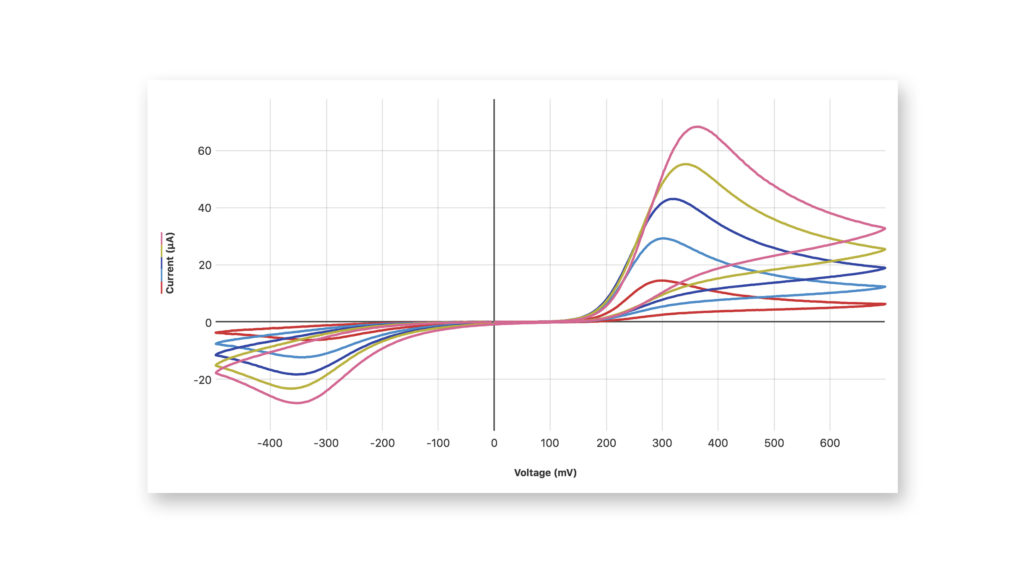# Analytical Chemistry

## Teach Your Students to Solve Complex Problems Across All Industries

Our integrated solution helps students collect accurate data, visualize trends and relationships, and explore different hypotheses for both conventional and innovative experiments.

##### QUALITY

For years, colleges and universities have relied on our durable hardware to help instructors teach key concepts.

##### AFFORDABLE

Our technology is designed and priced for student use.

##### VERSATILE
No matter what concepts you need to teach, Vernier technology can provide your students with practical, relevant data-collection and analysis experience.

## Example DataInvestigating cyclic voltammograms of acetaminophen at various concentrations using the Go Direct® Cyclic Voltammetry System and Vernier Instrumental Analysis™Examining the absorbance spectrum of potassium dichromate using the Vernier UV-VIS Spectrophotometer and Logger Pro®

This is only the beginning of what’s possible. See the recommendations below to get started with analytical chemistry.

Essential instructor information and word-processing files of student instructions are available to download for the following experiments.

## Featured Analytical Chemistry Experiments

### Spectrum of Atomic Hydrogen

You have no doubt been exposed many times to the Bohr model of the atom. You may have even learned of the connection between this model and bright line spectra emitted by excited gases. In this experiment, you will take a closer look at the relationship between the observed wavelengths in the hydrogen spectrum and the energies involved when electrons undergo transitions between energy levels.

### Planck’s Constant

The energy of a photon is related to its frequency by the equation E = hf, where h is Planck’s constant. By determining the potential required to excite an LED to emit light, you can estimate the energy of the photons emitted. Using a spectrometer, you can measure the peak wavelength of the emitted light; from this, the frequency can be calculated. Performing this analysis for a number of LEDs will enable you to obtain a reasonable approximation of the value of Planck’s constant.

### Acid-Base Titration

A titration is a process used to determine the volume of a solution needed to react with a given amount of another substance. In this experiment, you will titrate hydrochloric acid solution, HCl, with a basic sodium hydroxide solution, NaOH. The concentration of the NaOH solution is given and you will determine the unknown concentration of the HCl. Hydrogen ions from the HCl react with hydroxide ions from the NaOH in a one-to-one ratio to produce water in the overall reaction:${{\text{H}}^{\text{ + }}}{\text{(aq) + C}}{{\text{l}}^{\text{ - }}}{\text{(aq) + N}}{{\text{a}}^{\text{ + }}}{\text{(aq) + O}}{{\text{H}}^{\text{ - }}}{\text{(aq)}} \to {{\text{H}}_{\text{2}}}{\text{O(1) + N}}{{\text{a}}^{\text{ + }}}{\text{(aq) + C}}{{\text{l}}^{\text{ - }}}{\text{(aq)}}$

When an HCl solution is titrated with an NaOH solution, the pH of the acidic solution is initially low. As base is added, the change in pH is quite gradual until close to the equivalence point, when equimolar amounts of acid and base have been mixed. Near the equivalence point, the pH increases very rapidly. The change in pH then becomes more gradual again, before leveling off with the addition of excess base.

In this experiment, you will use a computer to monitor pH as you titrate. The region of most rapid pH change will then be used to determine the equivalence point. The volume of NaOH titrant used at the equivalence point will be used to determine the molarity of the HCl.

### Determining the Concentration of a Solution: Beer’s Law

The primary objective of this experiment is to determine the concentration of an unknown nickel (II) sulfate solution. To accomplish this, you will use a Colorimeter or a Spectrometer to pass light through the solution, striking a detector on the opposite side. The wavelength of light used should be one that is absorbed by the solution. The NiSO4 solution used in this experiment has a deep green color, so Colorimeter users will be instructed to use the red LED. Spectrometer users will determine an appropriate wavelength based on the absorbance spectrum of the solution. The light striking the detector is reported as absorbance or percent transmittance. A higher concentration of the colored solution absorbs more light (and transmits less) than a solution of lower concentration.

You are to prepare five nickel sulfate solutions of known concentration (standard solutions). Each is transferred to a small, rectangular cuvette that is placed into the Colorimeter or Spectrometer. The amount of light that penetrates the solution and strikes the detector is used to compute the absorbance of each solution. When a graph of absorbance vs. concentration is plotted for the standard solutions, a direct relationship should result. The direct relationship between absorbance and concentration for a solution is known as Beer’s law.

The concentration of an unknown NiSO4 solution is then determined by measuring its absorbance. By locating the absorbance of the unknown on the vertical axis of the graph, the corresponding concentration can be found on the horizontal axis. The concentration of the unknown can also be found using the slope of the Beer’s law curve.

• ### Go Direct® pH Sensor

$99.00$845.00